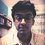# A hypothesis in Number Theory

Lately, while I was practicing for my JEE examination I discovered an amusing fact. I would like to share it with the Brilliant Community as a hypothesis.

## The Hypothesis

Given a number $p$, any number $N$ of the form:

1. $n(n-1)(n+1)(n-2)(n+2)\dots (n-\frac{p-1}{2})(n+\frac{p-1}{2})$ is always divisible by $p$ if $p$ is odd. $\left(n\in \text{natural numbers}\right)$

2. $n(n-1)(n+1)(n+2)(n-2)\dots (n -\frac{p}{2})$ is always divisible by $p$ if $p$ is even. $\left(n\in \text{natural numbers}\right)$

## My motivation towards the hypothesis

We know that any natural number $N$(if want to write it with respect to $p$) can be of only the following forms:$\{kp, kp+1, kp+2,\dots kp+p-1\}$ where $k$ is a whole number. Now I tried to write an expression for $N$ such that, no matter what form of number from the above set we put in that expression for $N$, it is always divisible by $p$ and that's how I got to those two expressions written above!

## So what's the use of this hypothesis

This hypothesis has helped me solve quite a number of problems in a sweet and simple fashion. It has at many places helped me reduce certain calculations. It certainly helps you solve problems where $N$ is given to you as a function of $n$ and you are asked to find the divisibilty of $N$ by a certain number. These are a fews ways where this hypothesis can be a bit resourceful!

## Conclusion

What I want from the Brilliant community is to help me come up with a more refined form of the two expressions if possible and some other amusing observation which when supplemented to this hypothesis can be highly useful. Do comment and share about how you felt about this note. Give as many inputs as possible, this will only help us all grow as a science community.

This was my first note and I will be highly grateful to the community if they provide feedbacks which can help me refine my note writing skills.

Thank you!Note by Miraj Shah
5 years, 3 months ago

This discussion board is a place to discuss our Daily Challenges and the math and science related to those challenges. Explanations are more than just a solution — they should explain the steps and thinking strategies that you used to obtain the solution. Comments should further the discussion of math and science.

When posting on Brilliant:

• Use the emojis to react to an explanation, whether you're congratulating a job well done , or just really confused .
• Ask specific questions about the challenge or the steps in somebody's explanation. Well-posed questions can add a lot to the discussion, but posting "I don't understand!" doesn't help anyone.
• Try to contribute something new to the discussion, whether it is an extension, generalization or other idea related to the challenge.

MarkdownAppears as
*italics* or _italics_ italics
**bold** or __bold__ bold
- bulleted- list
• bulleted
• list
1. numbered2. list
1. numbered
2. list
Note: you must add a full line of space before and after lists for them to show up correctly
paragraph 1paragraph 2

paragraph 1

paragraph 2

[example link](https://brilliant.org)example link
> This is a quote
This is a quote
    # I indented these lines
# 4 spaces, and now they show
# up as a code block.

print "hello world"
# I indented these lines
# 4 spaces, and now they show
# up as a code block.

print "hello world"
MathAppears as
Remember to wrap math in $$ ... $$ or $ ... $ to ensure proper formatting.
2 \times 3 $2 \times 3$
2^{34} $2^{34}$
a_{i-1} $a_{i-1}$
\frac{2}{3} $\frac{2}{3}$
\sqrt{2} $\sqrt{2}$
\sum_{i=1}^3 $\sum_{i=1}^3$
\sin \theta $\sin \theta$
\boxed{123} $\boxed{123}$

Sort by:

Your hypothesis is certainly correct, and a weaker version of the well known result that the product of n consecutive integers is divisible by n! .

- 5 years, 3 months ago